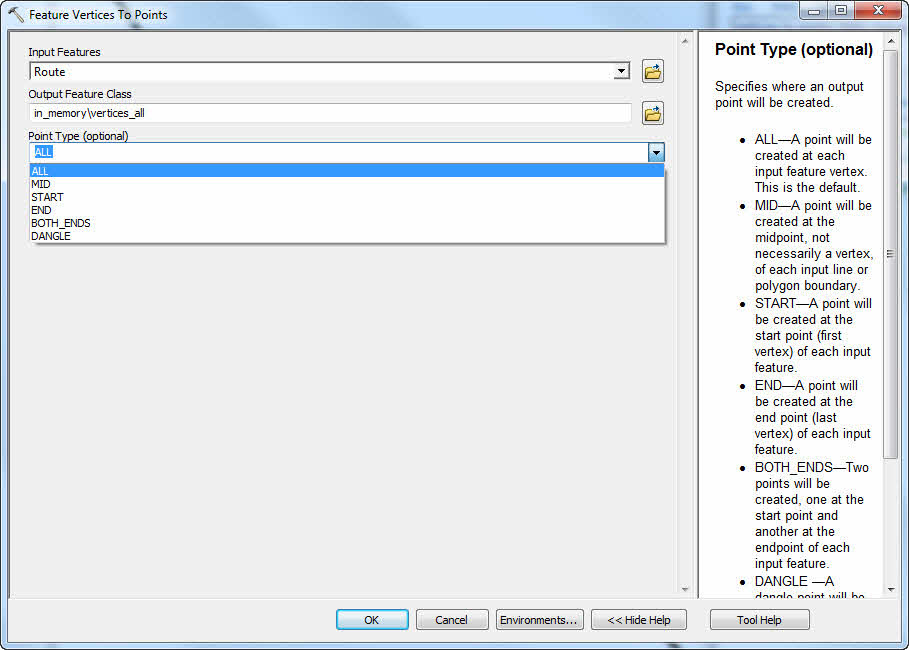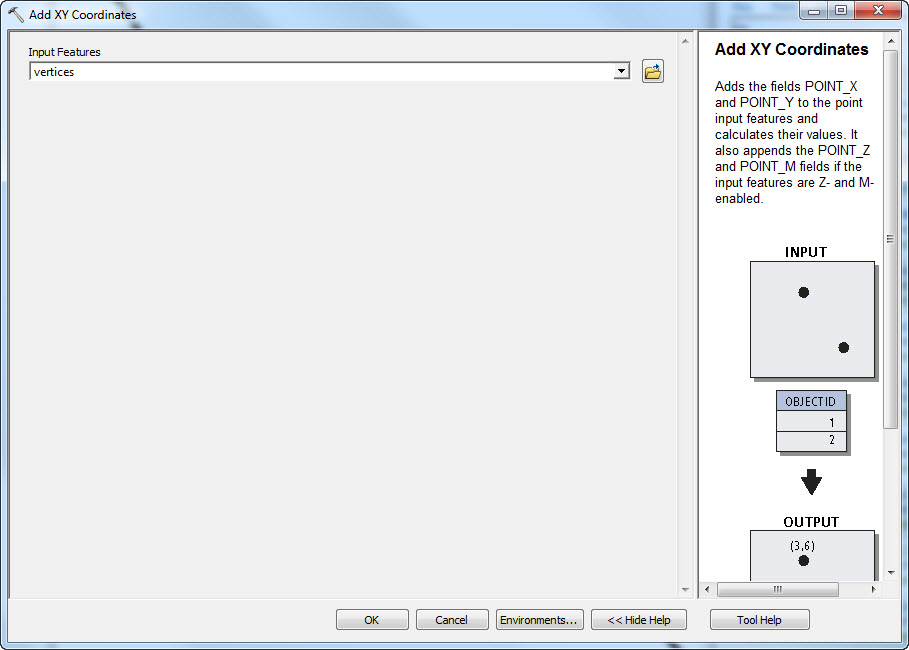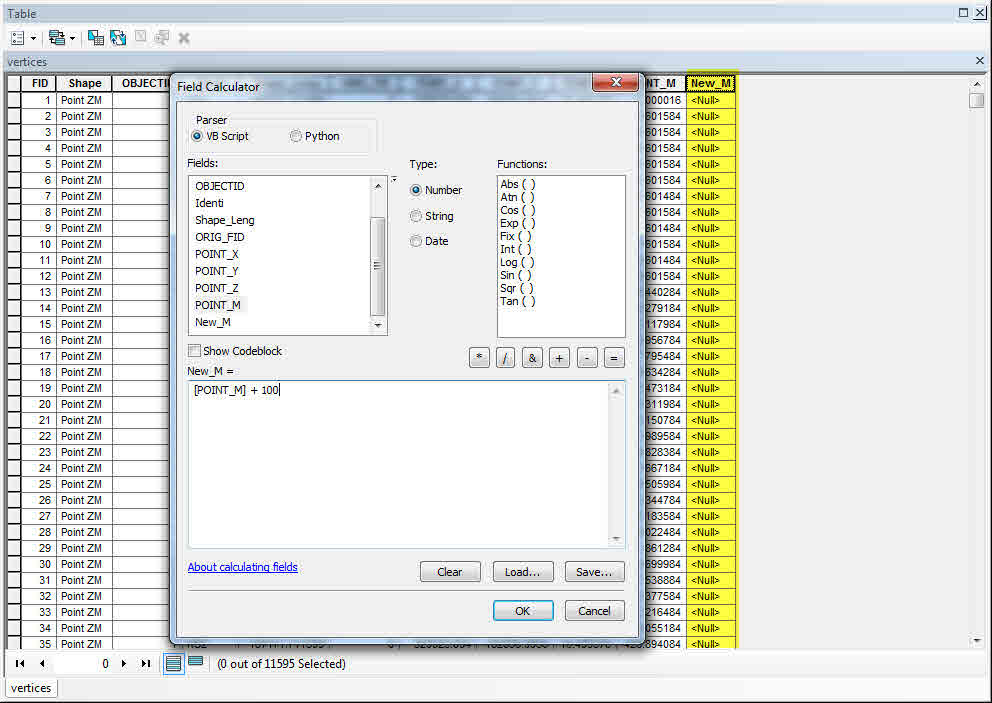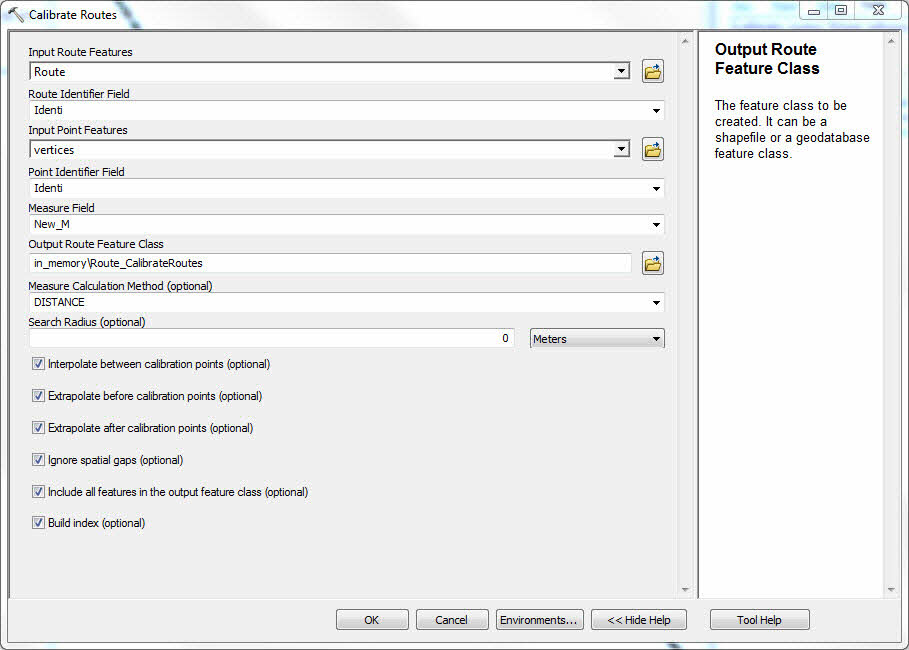English

# How To: Expose route M values (geometry) as attributes for route re-calibration

## Summary

M values, like X and Y, are stored for each vertex of a line. In order to use the Field Calculator to manipulate M values, the vertices must be converted to points, and the M values must be exposed in attribute format. Once the desired modifications have been made, the original routes can be calibrated using the new M values.

## Procedure

The following steps provide a general guideline for exposing, manipulating, and calibrating M values.

`Note:Some of the tools used in this workflow require an ArcInfo or ArcGIS for Desktop Advanced license.`

1. Use the Feature Vertices to Points tool to convert the route vertices to points. Depending on which vertices need to be modified, the ALL or BOTH_ENDS option could be used.
`Convert Vertices to Points`2. If the new M values are to be based on the existing M values, the M value for each point must be exposed as an attribute. Use the Add XY Coordinates tool to do this. This adds a POINT_X, POINT_Y, and POINT_M field to the points file.
`Add XY Coordinates`3. Add a field, and use the Field Calculator to generate the new M values.
`Calculate New Ms`4. Use the Calibrate Routes tool to calibrate the routes with the new M values. The Route Identifier Field and Point Identifier field should be the same (respectively), while the Measure Field should be the new field created and calculated in the previous steps.
`Calibrate Routes`